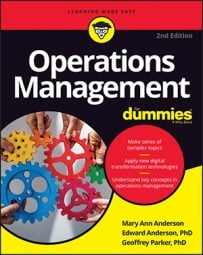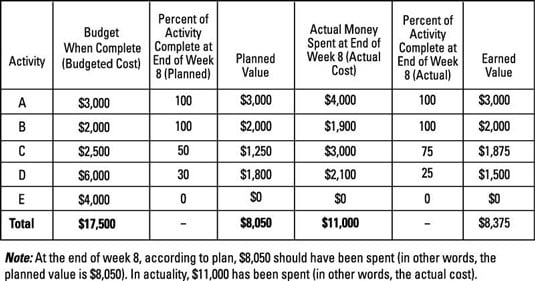##### Operations Management For DummiesThere are a number of ways to track the progress of your operations project. One of the simplest is to set up milestones during the project planning phase of the project life cycle. If there are enough milestones and they are spaced relatively evenly, you can get a good idea of where you are in the project by tracking which milestones have been completed on a regular basis.

A more sophisticated method is the earned value (EV) analysis approach. To use this approach, list all the activities in your project, along with their estimated costs.

To perform the analysis, find these four metrics for each activity:

• Budgeted cost: In the planning phase of the project, determine how much it will cost to complete each activity. For activity B, the budgeted cost is \$2,000. For activity D, it’s \$6,000. These values don’t change over time.

• Planned value: This is estimated from the planning phase upfront about how much you’ll spend on completing an activity. During the planning phase of the project, activity B was expected to be complete by the end of week 8. So 100 percent of the budgeted cost of \$2,000 yields a “planned value” of \$2,000.

In contrast, activity D was planned to be only 30 percent complete by end of week 8, so its planned value is 30 percent of the budgeted cost of \$6,000, which yields a planned value of \$1,800.

• Actual cost: This is what you actually end up spending on completing an activity. In the example, this is \$1,900 for activity B and \$2,100 for activity D. Usually, the actual cost won’t equal the planned value (although hopefully it’s close).

• Earned value: This is the actual percent complete for an activity times its budgeted cost. For activity B, 100 percent of the project was actually complete by the end of week 8; 100 percent of the budgeted cost for activity B of \$2,000 is \$2,000.

Thus, though it cost only \$1,900 to complete activity B, the earned value for project monitoring purposes is \$2,000. Activity D is only actually 25 percent complete at the end of week 8, so its earned value is 25 percent of its budgeted cost of \$6,000, or \$1,500. Note that activity D’s earned value will increase in subsequent weeks.Now you need to find the planned value (PV), actual cost (AC), and earned value (EV) of the entire project. To do this, you’ll figure out what activities you planned to complete by now in the project versus what you actually have completed. You’ll also need to figure out what you planned to spend versus what you actually have spent.

To find the PV for the project, sum up the PVs for all the activities at the end of week 8. This gives you a total of \$8,050. Adding up the ACs of all the activities totals \$11,000. Finally, summing the EVs totals \$8,375 at the end of week 8. This means that the PV is \$8,050, the AC \$11,000, and the EV is \$8,375 for this example project.

Now calculate these four measures of progress for the earned value method:

• Cost variance (CV): CV = EV – AC

This is how much less than you’ve spent by this point in the project than what you planned to. It’s measured in dollars. Counterintuitively, a positive variance is good, and a negative variance is bad.

• Schedule variance (SV): SV = EV – PV

This is how much more of the project you’ve completed by this point in the project than you planned to. It’s measured in dollars. Again, a positive variance is good; a negative variance is bad.

• Cost performance index (CPI): EV/AC

This is the ratio of how much you’ve spent on the project by this point over what you had planned to. It’s measured as a percentage. Counterintuitively, a value above 100 percent is good, and a value below 100 percent is bad.

• Schedule performance index (SPI): EV/PV

This is the ratio of how much of the project you’ve completed by this point over what you had planned to. It’s measured as a percentage. A value above 100 percent is good, and a value below 100 percent is bad.

In all four of these measures, higher is better. For example, a cost performance index (CPI) of 110 percent means that the project is not 10 percent over budget, but 9.09 percent under budget (1 – 1/1.10 = 0.909).

The example has these tracking measures:

• Cost variance is EV – AC = \$8,375 – \$11,000 = –\$2,625. This means the project is \$2,625 over budget at the end of week 8. Yes, negative does mean it’s over budget. A positive value would actually be under budget.

• Schedule variance is EV – PV = \$8,375 – \$8,050 = \$325. So the project is \$325 ahead of schedule at the end of week 8. (Yes, this method does use dollars to measure time!)

• Cost performance index is EV/AC = \$8,375/\$11,000 = 76.1 percent. This means the project is significantly over budget. (1/0.761 – 1 = 31.3 percent over budget, to be precise.) Frankly, for project management purposes, saying that the project is about 100 percent – 76 percent = 34 percent over budget is close enough.

• Schedule performance index is EV/PV = \$8,375/\$8,050 = 104 percent, which means that you are 4 percent ahead of schedule at the end of week 8.

Based on this earned value analysis, your project at week 8 is a little ahead of schedule. Unfortunately, it is way over budget.

Mary Ann Anderson is Director of the Supply Chain Management Center of Excellence at the University of Texas at Austin.

Edward Anderson, PhD, is Professor of Operations Management at the University of Texas McCombs School of Business.

Geoffrey Parker, PhD, is Professor of Engineering at Dartmouth College.

Mary Ann Anderson is Director of the Supply Chain Management Center of Excellence at the University of Texas at Austin.

Edward Anderson, PhD, is Professor of Operations Management at the University of Texas McCombs School of Business.

Geoffrey Parker, PhD, is Professor of Engineering at Dartmouth College.

Mary Ann Anderson is Director of the Supply Chain Management Center of Excellence at the University of Texas at Austin.

Edward Anderson, PhD, is Professor of Operations Management at the University of Texas McCombs School of Business.

Geoffrey Parker, PhD, is Professor of Engineering at Dartmouth College.